Students must start practicing the questions from CBSE Sample Papers for Class 10 Maths with Solutions Set 10 are designed as per the revised syllabus.

## CBSE Sample Papers for Class 10 Maths Standard Set 10 with Solutions

Time Allowed: 3 Hours
Maximum Marks: 80

General Instructions:

1. This Question Paper has 5 Sections A-E.
2. Section A has 20 MCQs carrying 1 mark each
3. Section B has 5 questions carrying 02 marks each.
4. Section C has 6 questions carrying 03 marks each.
5. Section D has 4 questions carrying 05 marks each.
6. Section E has 3 case based integrated units of assessment (04 marks each) with sub-parts of the values of 1, 1 and 2 marks each respectively.
7. All Questions are compulsory. However, an internal choice in 2 Qs of 5 marks, 2 Qs of 3 marks and 2 Questions of 2 marks has been provided. An internal choice has been provided in the 2 marks questions of Section E
8. Draw neat figures wherever required. Take π =22/7 wherever required if not stated.

Section – A
(Section A consists of 20 questions of 1 mark each.)

Questions 1.
If one root of the equation 2x2 – 5x +(k – 6) = 0 be the reciprocal of the other, then: (1)
(a) k = -8
(b) k=8
(c) k = 4
(d) k = -4
(b) k = 8

Explanation:
Given, equation is 2x2 – 5x + (k – 6) = 0
Let, the roots be α and $$\frac{1}{α}$$
Product of roots = $$\frac{k-6}{2}$$
⇒ α × $$\frac{1}{α}$$ = $$\frac{k-6}{2}$$
$$\frac{k-6}{2}$$ = 1
⇒ k – 6 = 2
⇒ k =8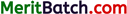Questions 2.
A circle on the coordinate axes, such that the origin is the centre of the circle and it passes though ($$\frac{13}{2}$$,0). The point which does not lie in the interior of the circle is:
(a) ($$-\frac{13}{2}$$,1)
(b) (2, $$\frac{7}{3}$$)
(c) (5, $$-\frac{1}{2}$$)
(d) (-6, $$\frac{5}{2}$$)
(d) (-6, $$\frac{5}{2}$$)

Explanation:
Since, the centre of the circle is at the origin (0, 0) and it passes through the point ($$\frac{13}{2}$$,0)
This, radius of the circle = $$\sqrt{\left(\frac{13}{2}-0\right)^2+(0-0)^2}$$
= $$\frac{13}{2}$$ units = 6.5 units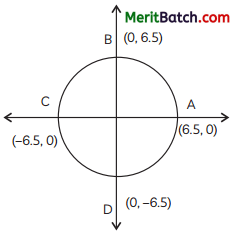Distance between (0, 0) and ($$\frac{-3}{4}$$,1)
= $$\sqrt{\left(\frac{-3}{4}-0\right)^2+(1-0)^2}$$
= $$\sqrt{\left(\frac{9}{16}-0\right)+1}$$
= $$\sqrt{\frac{25}{10}}$$
= $$\frac{5}{4}$$ units = 1.25 units

So, the point ($$\frac{-3}{4}$$,1) lies in the interior of the circle.
Distance between (0, 0) and (2, $$\frac{7}{3}$$)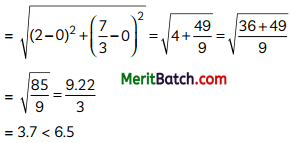So, the point (2, $$\frac{7}{3}$$) lies in the interior of the circle.
Distance between (0, 0) and (5, –$$\frac{1}{2}$$)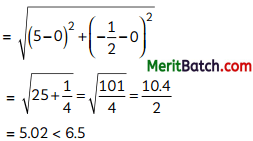So, the point lies in the interior of the circle.
Distance between (0, 0) and (-6, $$\frac{5}{2}$$)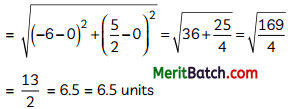So this point lies on the circle not in the interior.

Question 3.
In the following distribution:

 Marks obtained Number of students More than or equal to 0 63 More than or equal to 10 58 More than or equal to 20 55 More than or equal to 30 51 More than or equal to 40 48 More than or equal to 50 42

The frequency of the class 30 – 40 is:
(a) 3
(b) 4
(c) 48
(d) 51
(a) 3

Explanation:
Given distribution is:

 Marks obtained Number of students frequency 0-10 63 – 58 = 5 5 10-20 58 – 55 = 3 3 20-30 55 – 51 = 4 4 30-40 51 – 48 = 3 3 40-50 48 – 42 = 6 6 50-60 42 42

Hence frequency of class 30-40 is 3.

Question 4.
The greatest number which divides 70 and 125, leaving remainders 5 and 8, respectively is:
(a) 13
(b) 65
(c) 875
(d) 1750
(a) 13

Explanation:
Number when divides 70 and 125 leaves 5 and 8 as remainder.
Then,
70 – 5 = 65
125 – 8 = 117
HCF of 65 and 117
65 = 5 × 13
117 = 3 × 3 × 13
HCF is 13
Hence, the required number is 13.Question 5.
If the first term of an AP is -5 and the common difference is 2, then the sum of the first 6 terms is:
(a) 0
(b) 5
(c) 6
(d) 15
(a) 0

Explanation:
Given, first term of AP, a = -5 common difference, d = 2
Sum of first 6 terms
n
Sn = $$\frac{n}{2}$$[2° + (n – 1)d]
S6 = $$\frac{6}{2}$$[2 × (-5) + (6 -1) × 2]
= 3(-10 + 10)
= 0

Question 6.
If 2 cos 3A = 1, then the value of A is:
(a) 20°
(b) 30°
(c) 60°
(d) 45°
(a) 20°

Explanation:
2 cos 3A = 1
cos 3A = $$\frac{1}{2}$$
⇒ cos 3A = cos 60° [cos 60° = $$\frac{1}{2}$$]
⇒ 3A = 60°
⇒ A = 20°

Question 7.
AABC is not similar to ADEF but ∆ABC ~ ∆EDF, then which of the following is incorrect?
(a) BC. EF = AC. FD
(b) AB. EF = AC. DE
(c) BC. DE = AB. EF
(d) BC. DE = AB. FD
(c) BC. DE = AB. EF

Explanation:
∆ABC ~ ∆EDF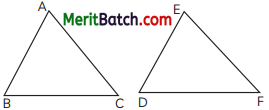Since, corresponding sides of similar ∆S are proportional
$$\frac{A B}{E D}=\frac{B C}{D F}=\frac{C A}{F E}$$
Then only (c) option is not correct.

Question 8.
The value of k, for which the pair of linear equations 3x – y + 8 = 0 and 6x – ky + 16 = 0 have infintely many solution is:
(a) $$\frac{1}{2}$$
(b) –$$\frac{1}{2}$$
(c) -2
(d) 2
(d) 2

Explanation: Here, equations are :
3x – y + 8 = 0 and 6x – ky + 16 = 0
On compairing the given equations with
a1x + b1y + c1 = 0
and a2x + b2y + c2 = 0 we get,
a1 = 3, b1 = -1, ci1 = 8
and a2 = 6, b2 = -k, c2 = 16
$$\frac{a_1}{a_2}=\frac{b_1}{b_2}=\frac{c_1}{c_2}$$
$$\frac{3}{6}=\frac{-1}{-k}=\frac{8}{16}$$
k = 2Question 9.
If Σfi = 11, Σfixi = 2p + 52 and the mean of distribution is 6, then the value of p is:
(a) 7
(b) 8
(c) 9
(d) 18
(a) 7

Explanation:
Σfi = 11
Σfixi = 2p + 52
$$\bar{x} = 6$$
$$\bar{x}=\frac{\sum f_i x_i}{\sum f_i}$$
6 = $$\frac{2p+52}{11}$$
2p + 52 = 66
2p = 66 – 52
2p = 14
p = 7

Question 10.
If the zeroes of the quadratic polynomial x2 + (p + 1)x + q are 2 and -3, then:
(a) p = -7,q = -1
(b) p = 5, q = -1
(c) p = 2, q = -6
(d) p = 0, q = -6
(d) p = 0, q = -6

Explanation:
Here, polynomial is : x2 + (p + 1)x + q
Zeroes of polynomial are 2 and – 3
sum of zeroes = $$\frac{-b}{a}$$ = $$\frac{-(p+1)}{1}$$= 2 – 3
p + 1 = 1
p = 0
Product of zeroes = $$\frac{c}{a}$$ = $$\frac{q}{1}$$
q = 2 × (-3) = -6

Question 11.
The sum of the three sides of a triangle whose vertices are (0,0), (0,4) and (3,0) is:
(a) 12 units
(b) 5 units
(c) 11 units
(d) (7 + $$\sqrt{5}$$)units
(a) 12 units

Explanation:
Sum of 3 sides = OA + AB + OB
By distance formula
= \begin{aligned} \sqrt{(4-0)^2+(0-0)^2}+\sqrt{(4-0)^2+(0-3)^2} \\ &+\sqrt{(0-0)^2+(3-0)^2} \end{aligned}
= 4 + 5 + 3
= 12 units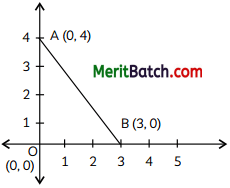Question 12.
A mason constructs a wall of dimensions 270 cm × 300 cm × 350 cm with the bricks each of size 22.5 cm × 11.25 cm × 8.75 cm
and it is assumed that $$\frac{1}{8}$$ space is covered 8 with mortar. How many bricks are used to construct the wall ?
(a) 11,000
(b) 11,100
(c) 11,200
(d) 11,300
c) 11,200

Explanation:
Volume of the wall = 270 × 300 × 350 = 28350000 cm3
Volume of a brick = 22.5 × 11.25 × 8.75 = 2214.84375cm3

Volume of wall covered by mortar 1
= -× 270 × 300 × 350
=135 × 75 × 350
= 3543750 cm3

Volume of wall covered by bricks
=2,83,50,000 – 35,43,750
= 2,48,06,250 cm3

Let number of bricks used be n
A.T.Q.
n × 22.5 × 11.25 × 8.75 = 2,48,06,250
n = 11,200

Question 13.
Seema purchased a lottery ticket. She was very curious to know her winning chances. The probability of her winning the first prize in a lottery is 0.08. If 6000 tickets are sold, how many tickets has she bought?
(a) 40
(b) 240
(c) 480
(d) 750
(c) 480

Explanation:
Total tickets sold = 6000 Probability that Seema will win = 0.08
P(of seema winning) = $$\frac{Tickets she bought }{Total tickes sold}$$
Tickets she bought = 6000 × 0.08 = 480

Question 14.
A pair of tangents is drawn on a circle from an external point. If these tangents are perpendicular to each other and each is of length 5 cm, then the radius of the circle is:
(a) 10 cm
(b) 7.5 cm
(c) 5 cm
(d) 2.5 cm
(c) 5 cm

Explanation:
Here,
∠OAB = 90° (Tangent is ⊥r to radius at point of contact)∠OBA = 45° (Tangents are inclined equally on OB)
In ∆OAB
tan 45° = $$\frac{OA}{BA}$$ = $$\frac{OA}{5}$$
OA = 5 cm

Question 15.
The 4th term from the end of the AP: -10, -8, -6,…………… 48 is:
(a) 37
(b) 42
(c) 43
(d) 58
(b) 42

Explanation:
Given, AP is -10, -8, -6, ………… 48
Now, series, : 48 -6, -8, -10
common difference, d = -8 – (-6)
= -2
Then, a4 = a + 3d
= 48 + 3 × (-2)
= 48 – 6
= 42Question 16.
If triangles ∆ABC and ∆DEF, $$\frac{A B}{E F}=\frac{B C}{E D}=\frac{C A}{D F}$$ then:
(a) ∆DEF ~ ∆CAB
(b) ∆DEF ~ ∆ABC
(c) ∆CBA ~ ∆DEF
(d) ∆BCA ~ ∆DEF
(a) ∆DEF ~ ∆CAB

Explanation:
In ∆s ABC and DEF
$$\frac{A B}{E F}=\frac{B C}{P D}=\frac{C A}{D F}$$
∴ ∆ABC ~ ∆EFD
or ∆CAB ~ ∆DEF

Question 17.
A quadratic polynomial, whose zeroes are -2 and 4 is:
(a) x2 – 2x – 8
(b) x2 – 2x + 4
(c) 2x2 – x – 4
(d) x2 + 2x – 8
(a) x2 – 2x – 8

Explanation:
Given, zeros are – 2 and 4
Sum of zeroes = 2
Product of zeroes = – 8
∴ Quadratic polynomial = x2 – (sum of zeroes)x + Product of zeroes
= x2 – 2x – 8

Question 18.
A school divides its sutdents into 5 houses A, B, C, D and E. Class XA has 23 students, 4 from house A, 8 from house B, 5 from house C, 2 from house D and rest from house E. A single student is chosen at random to become the monitor of the class. The probability that the chosen student is not from houses A, B and C is:
(a) $$\frac{4}{23}$$
(b) $$\frac{6}{23}$$
(c) $$\frac{8}{23}$$
(d) $$\frac{17}{23}$$
(b) $$\frac{6}{23}$$

Explanation:
Total students in class XA = 23
students from house A = 4
students from house B = 8
students from house C = 5
students from house D = 2
students from house E = 4

Total students in house D and E = 2 + 4 = 6
Required probability = $$\frac{6}{23}$$

DIRECTION: In the question number 19 and,, 20, a statement of assertion (A) is followed by a statement of reason (R).
Choose the correct option as:
(a) Both assertion (A) and reason (R) are true and reason (R) is the correct explanation of assertion (A)
(b) Both assertion (A) and reason (R) are true and reason (R) is not the correct explanation of assertion (A)
(c) Assertion (A) is true but reason (R) is false.
(d) Assertion (A) is false but reason (R) is true.

Question 19.
Statement A (Assertion): If k = $$\frac{1}{2}$$, then the points (k, 2 – 2k), (-k + 1, 2k) and (-4 – k, 6 – 2k) are collinear
Statement R (Reason): Three points P, Q and k are collinear in the same straight line if PQ + QR = PR.
(a) Both assertion (A) and reason (R) are true and reason (R) is the correct explanation of assertion (A).

Explanation:
Where more than three points are collinear slope of the line are same.
So,
Slope : [(k,2 – 2k), [(-k + 1, 2k)] = [(-k + 1, 2k),(-4 -k, 6 – 2k)]
$$\frac{2-2k-2k}{k+k-1}=\frac{2k-6+2k}{-k+1+4+k}$$
$$\frac{2-4k}{2k-1}=\frac{4x-6}{5}$$
(1 – 2k) 5 = (2k – 3) (2k – 1)
5 – 10k = 4k2 – 2k – 6k + 3
4k2 + 2k – 2 = 0
2k2 + k – 1 = 0
2k2 + 2k – k – 1= 0
2k(k + 1) – 1 (k + 1) = 0
(2k – 1)(k + 1) = 0
k = -1 or $$\frac{1}{2}$$

Question 20.
Statement A (Assertion): The external dimensions of a wooden box are 18 cm, 10 cm and 6 cm respectively and thickness of the wood is 5 mm, then the internal volume is 765 cm3.
Statement R (Reason): If external dimensions of a rectangular box t, b and ft and the thickness of the sides be x, then its internal volume is (l – 2x)(b -2x) (h – 2x).
(a) Both assertion (A) and reason (R) are true and reason (R) is the correct explanation of assertion (A).

Explanation:
Length of box = 18 cm
width of box = 10 cm
height of box = 18 cm
thickness of box = 5 mm = $$\frac{1}{2}$$ cm

Internal lenth, width and height of the box is
$$\left(18-\frac{2 \times 1}{2}\right),\left(10-\frac{2 \times 1}{2}\right),\left(6-\frac{2 \times 1}{2}\right)$$
Internal volume of box = 17 × 9 × 5 = 765 cm2Section – B
(Section B consists of 5 questions of 2 mark each.)

Question 21.
A light house is used to used to warn mariners of dangerous shallows rocky coasts. Light house in a sea can throw the light up to a distance of 70 m. If the angle it makes while spreading light is 60°, find the area covered by it.
When a light house spreads light, it forms a sector of angle 60° and radius 70 cm.
Area of sector = $$\frac{\theta}{360^{\circ}} \times \pi r^2$$
= $$\frac{1}{6} \times \frac{22}{7} \times 70 \times 70$$
= $$\frac{1}{6} \times 700$$
= $$\frac{7700}{3}$$ m2
Hence, light will be spread in the area of $$\frac{7700}{3}$$ m2

Question 22.
Find the 10th, 23rd and 30th terms of the AP: 9, 13, 17, 21, ………..
OR
An envelope contains 40 shopping vouchers, of which 25 vouchers each has a value of ₹ 100 and 15 vouchers each has a value of ₹ 500. Anjana picks up a voucher it random from the envelope. Find the probability that the voucher has a value of
(A) ₹ 500
(B) ₹ 50
Given, AP is 9, 13, 17, 21, ………..
Here, first term, a = 9
common difference, d = 13 – 9 = 4

Then, a10 = a + (n – 1)d
= 9 + (10 – 1) × 4
= 9 + 36
= 45

a23 = 9 + (23 – 1) × 4
= 9 + 22 × 4
= 9 + 88
= 97

a30 = 9 + (30 – 1) × 4
= 9 + 29 × 4
= 9 + 116
= 125

OR

Total number of shopping vouchers = 40
No. of vouchers of value of ₹ 100 = 25
No. of vouchers of value of ₹ 500 = 15

(A) P(getting a voucher of ₹ 500) = $$\frac{15}{40}$$
= $$\frac{3}{8}$$
(B) P(getting a voucher of ₹ 50) = $$\frac{0}{40}$$
= 0Question 23.
Draw the graph of the quadratic polynomial p(x) = 3 – 2x – x2.
Let p(x) = 3 – 2x – x2.

 x -5 -4 -3 -2 -1 0 1 2 3 4 y -12 -5 0 3 4 3 0 -5 -12 -21

There are few values of y corresponding to x.Question 24.
If 5 cos 0 = 3, find the value of $$\sqrt{\frac{1-\sin \theta}{1+\sin \theta}}$$
Given:
5 cos θ = 3
cos θ = $$\frac{3}{5}$$ = $$\frac{B}{H}$$

Now, P2 + B2 = H2
(by Pythagoras theorem)
p2 = H2 – B2
= 52 – 32 = 25 – 9 = 16
P = 4 units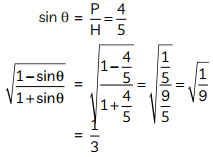Question 25.
Show graphically that the following pair of linear equations has infinitely many solutions:
3x – y = 2; 9x – 3y = 6.
OR
Find the roots of the following equation by using quadratic formula: 2x2 + 6$$\sqrt{3}$$x – 60 = 0
Given, pair of Linear equations are,
3x – y – 2 = 0 …(i)
and 9x – 3y – 6 = 0 …(ii)
For equation (i),
y = 3x – 2

 x 0 1 2 y -2 1 4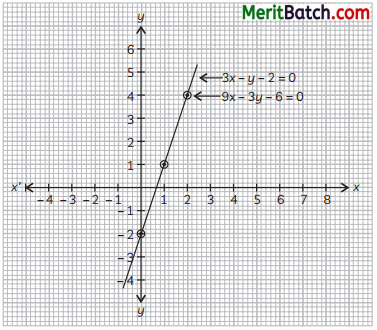for equation (ii)
y = $$\frac{9x=6}{3}$$

 x 0 1 2 y -2 1 4

Both the equations are represented by the same Line So they overlap. Hence there are infinitely many solutions.
OR
The given equation is 2x2 + 6$$\sqrt{3}$$x – 60 = 0 comparing it with standard form of equation ax2 + bx + c = 0, we get
a = 2, b = 6$$\sqrt{3}$$, c = -60
D = b2 – 4ac = (6$$\sqrt{3}$$)2 – 4 × 2 × (-60)
= 108 + 480 = 588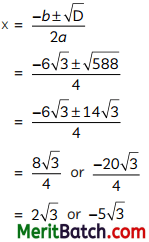Hence, roots are 2$$\sqrt{3}$$ and -5$$\sqrt{3}$$Section – C
(Section C consists of 6 questions of 3 mark each.)

Question 26.
For the first three days of a lending library, there is a fixed charge, and then there is an additional charge for each consecutive day. For a book that she kept for seven days, Saritha paid ₹ 27, while Susy paid ₹ 21 for a book she kept for five days. Find the fixed charge and the charge for each extra day.
Let fixed charge for 3 days = ₹ x
Let additional charge for each day thereafter = ₹ y
According to given condition, we have
x + 4y = 27 …(i)
x + 2y = 21 …(ii)
Subtracting (ii) from (i), we get
2y = 6
⇒ y = 3
Putting value of y in (i), we get
x + 4(3) = 27
⇒ x = 27 – 12 = 15
Therefore, fixed charge for 3 days = ₹ 15 and
additional charge for each day after 3 days = ₹ 3

Question 27.
A rectangular cardboard of dimensions 14 cm × 7 cm is taken. Two circular pieces of equal radii and maximum area, touching each other are cut out from it. Find the area of the remaining cardboard.
Here, ABCD is a cardboard of dimensions 14 cm and 7 cm.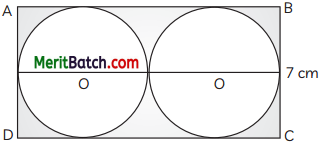Two circles of centre O and O’ are cut from the cardboard.
Diameter of each circle = 7 cm
Radius (r) of each circle = 3.5 cm
∴ Area of remaining cardboard = Area of cardboard – Area of 2 circles
= 14 × 7 – 2 x π (r2)
= 98 – 2 × $$\frac{22}{7}$$ × $$\frac{22}{7}$$ × $$\frac{22}{7}$$
= 98 – 77
= 21 sq. cm

Question 28.
Find the value of k, if-(a, b) is the mid¬point of the line segment joining the points A(10, -6) and B(k, 4) and a – 2b = 18. Also, find the distance AB.
OR
In the given figure, CD and GH are respectively the medians of ∆ABC and ∆EFG. If ∆ABC ~ ∆FEG, then prove that ∆ADC ~ ∆FHG(a, b) is the mid-point of Line segment joining the points A(10, -6) and B(k, 4)
So, a =$$\frac{10+k}{2}$$ and b = $$\frac{-6+4}{2}$$ = -1
It is given that,
a – 2b = 18
Putting values of a and b
$$\frac{10+k}{2}$$ – 2(-1) = 18
$$\frac{10+k}{2}$$ = 16
k = 22

∴ Distance between the points A(10, -6) and B(22, 4) is:
AB = $$\sqrt{(22 -10)^2 + (4 + 6)^2}$$
= $$\sqrt{244}$$
= $$2 \sqrt{61}$$ units

ORAnd ∆ABC ~ ∆EFG
Proof: Since, ∆ABC ~ ∆EFG and CD and GH are medians of ∆s.Question 29.
A ∆ABC is such that, the points are on the side AC and BC and DE || AB and EF || BD. Prove that DC2 = CF × AC.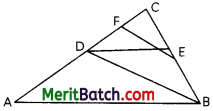Given: In AABC,
DE || AB and EF || BDTo Prove: DC2 = CF × AC
Proof: In AABC, as DE || AB
∴ $$\frac{C D}{A D}=\frac{C E}{E B}$$ ……(i)
and in ACDB, as EF || BD
∴ $$\frac{C F}{F D}=\frac{C E}{B E}$$
From (i) and (ii)
$$\frac{C D}{A D}=\frac{C F}{F D}$$
or $$\frac{A D}{C D}=\frac{F D}{C F}$$
$$\frac{A D}{C D}+1=\frac{F D}{C F}+1$$
$$\frac{A D+C D}{C D}=\frac{F D+ C F}{C F}$$
$$\frac{AC}{DC}=\frac{CD}{C F}$$
DC2 = CF × AC
Hence, ProvedQuestion 30.
Inscribed in a right circular cone are two spheres with radii of 6 cm and 1 cm. The larger sphere touches both the smaller sphere and the cone’s base. Find the cone’s height.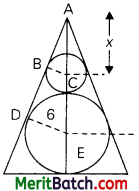The number of days 30 workers of a factory were absent in a year was found to be as below:
2, 14, 50, 72, 9, 48, 61, 60, 75, 39, 4, 8,
26, 62, 70, 58, 29, 77, 64, 43, 56, 67,
45, 58, 69, 76, 6, 50, 17, 66.
Prepare the frequency table with overlapping intervals of size 10, and calculate the mean of the number of days the workers were absent. (3)
Here As ABC and ADE are similar
∴ $$\frac{6}{1}$$ = $$\frac{x+1+6}{x}$$
⇒ 6x = x + 7
⇒ 5x = 7
x = $$\frac{7}{5}$$
∴ Height of the cone = x + 1 + 12
= x + 13
= $$\frac{7}{5}$$ + 13
= $$\frac{72}{5}$$ cm

OR

Here smallest value is 2.
Greatest value is 77.
Range = 77 – 2 = 75.
No.of classes = $$\frac{75}{10}$$ = 7.5 = 8
∴ Frequency distribution is:Mean $$\bar { x }$$ = $$\frac{\sum f_i x_i}{\sum f_i}$$
= $$\frac{1380}{30}$$ = 46
Hence the required mean is 46.

Question 31.
A class of 38 students went to a trip to Singapore. Out of the 18 boys , 6 of them checked in their language at the airport. 8 of the girls did not check in their language. If a student is chosen at random, find the probability that the student:
(A) is a girl who did not check in her language
(B) checked in his/her language. (3)
Total number of students went on the trip = 38
No. of boys = 18
No. of girls = 38 – 18 = 20
Boys checked in their Language = 6
Boys did not checked in their Language
18 – 6 = 12
GirLs did not cheked in their Language = 8
Girls checked in their language = 20 – 8 = 12
(A) No. of girls checked in their Language = 12
Required probability = $$\frac{12}{38}$$ = $$\frac{6}{19}$$

(B) No. of girls and boys checked in their language = 6 + 12
= 18
Required probability = $$\frac{18}{38}$$ = $$\frac{9}{19}$$

Section – D
(Section D consists of 4 questions of 5 mark each.)

Question 32.
In a flight of 60 km, an aeroplane was slowed down due to bad weather. Its average speed for the trip was reduced by 200 km/h and the time of flight increased by 30 minutes. Find the original duration of the flight. (5)
Let, the original speed of the aeroplane be x km/hr.
Then, new speed = (x – 200) km/hr.

Duration of flight at original speed = $$\frac{600}{x}$$
Duration of flight at reduced speed = ($$\frac{600}{x-200}$$)hr
∴ $$\frac{600}{x-200}$$ – $$\frac{600}{x}$$ = $$\frac{1}{2}$$
⇒ $$\frac{600-600(x-200)}{x(x-200)}$$ = $$\frac{1}{2}$$
⇒ $$\frac{120000}{(x^2-200x)}$$ = $$\frac{1}{2}$$
⇒ x2 – 200x – 240000 = 0
⇒ x2 – 600x + 400x – 240000 = 0
⇒ x (x – 600) + 400 (x – 600) = 0
⇒ (x – 600) (x + 400) = 0
⇒ x = 600 or x = -400 (not possible)
So, original speed of aeroplane was 600
∴ Duration of flight = $$\frac{600}{600}$$ = 1hr.
600
Hence, originial duration of flight is 1 hr.Question 33.
If cosec θ – sin θ = p and sec θ – cos θ = q, then prove that
(A) p2 q2(p2 + q2 + 3) = 1
(B) (p2 q)2/3 + (pq2)2/3 = 1.
OR
Two stations are located at a distance of “a” and “b” from the foot of a leaning tower that leans in the direction of the north. If a and b be the elevations of the top of the tower from these stations, show that the inclination q to the horizontal is given by $$\cot \theta=\frac{b \cot \alpha-a \cot \beta}{b-a}$$
Given:
cosec θ – sin θ = p(A) L.H.S. = p2 q2(p2 + q2 + 3)Hence, Proved.

(B) L.H.S. = (p2 q)2/3 + (pq2)2/3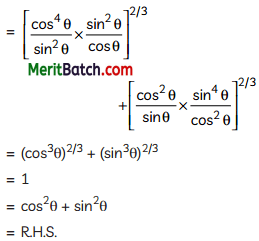Hence, Proved.

OR

Let, the height of the tower DE = h
Distance between first station to foot of tower AD = a + x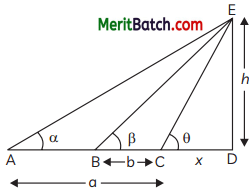Distance between second station to foot of tower BD = b + x
Distance between C and D = x
Given, a and b are angles of elevation of two station to the top of the tower.
i.e. ∠DAE = α, ∠DBE = ß, ∠DCE = θ
In ∆CDE,
cot θ = $$\frac{x}{h}$$ …(i)

In ∆BDE,
cot ß = $$\frac{b + x}{h}$$
b + x = h cot ß …….(ii)
Multiply ‘a’ on both sides
ab + ax = ha cot ß …(ii)

cot α = $$\frac{a + x}{h}$$
a + x = h cot α
Multipity ‘b’ on both sides
ba + bx = bh cot α …(iii)
Subtract (iii) from (ii)
(b – a)x = h (b cot α – a cot ß)
$$\frac{x}{h}$$ = $$\frac{b cot α – a cot ß}{b-a}$$
cot θ = $$\frac{b cot α – a cot ß}{b-a}$$ [from (i)]
Hence, proved.Question 34.
Three sets of Science, S.St and Computer books have to put in such a way that all the books are stored subject – wise and the height of each stack is the same. The number of science books are 96, the number of S.St books are 240 and the number of Computer books are 336. If we assume that the books are of the same thickness, determine the number of stacks of Science, S.St and Computer books. (5)

No. of stacks of Science books = 96
No. of stacks of S. St books = 240
No. of stacks of Computer books = 336
For finding the number of books the each stock, we find the HCF of 96, 240 and 336
Prime factor of 96, 240 and 336 are:
96 = 25 x 3
240 = 24 × 3 × 5
336 = 24 × 3 × 7
HCF = 24 × 3 = 48

No. of stacks of Science books = $$\frac{96}{48}$$ = 2
No. of stacks of S. St books = $$\frac{240}{48}$$ = 5
No. of stacks of Computer books = $$\frac{336}{48}$$ = 7
∴ Total no. of stacks = 2 + 5 + 7 = 14

Question 35.
A circle with the centre O has been inscribed with the quadrilateral ABCD. CD is produced to E in a way that ∠AED = 95° and ∠OBA=30°. Find ∠OAC.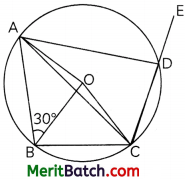OR
The following frequency distribution gives the monthly consumption of electricity of 68 consumers of a locality. Find the median, mean and mode of the data and compare them.

 Monthly consumption No. of consumers 65 – 85 4 85 – 105 5 105 – 125 13 125 – 145 20 145 – 165 14 165 – 185 8 185 – 205 4

In the figure, ABCD is a cyclic quadrilateral CD is produced to E such that ∠ADE = 95°. O is the centre of the circle.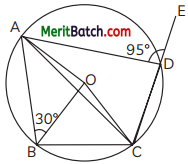⇒ ∠ADC + 95° = 180°
⇒ ∠ADC = 180° – 95° = 85°
Now arc ABC subtends ∠AOC at the centre and ∠ADC at the remaining part of the circle.
∴ ∠AOC = 2∠ADC = 2 × 85° = 170°
Now in DOAC,
∴ ∠OAC + ∠OCA + ∠AOC = 180°
(Sum of angles of a triangle)
∠OAC = ∠OCA
(∵ OA = OC radii of circle)
∴ ∠OAC + ∠OAC + 170° = 180°
2∠OAC = 180° – 170° = 10°
∴ ∠OAC = $$\frac{10°}{2}$$ = 5°

OR

 Monthly consumption No. of consumers (fi) x fixi c.f 65 – 85 4 75 300 4 85 – 105 5 95 475 9 105 – 125 13 115 1495 22 125 – 145 20 135 2700 42 145 – 165 14 155 2170 56 165 – 185 8 175 1400 64 185 – 205 4 195 780 68 Σfi=68 Σfixi=9320

For mean: Mean = $$\frac{\Sigma f_i x_i}{\Sigma f_i}=\frac{9320}{68}=137.05$$

For median: n = 68 and $$\frac{n}{2}$$ = 34
Median class = 125 -145
we have, l = 125, cf = 22, f = 20 and h = 20
Median = $$l+\left(\frac{\frac{n}{2}-c f}{f}\right) \times h$$
= $$125+\left(\frac{34-22}{20}\right) \times 20$$
= 125 + 12
= 137

For mode:
Modal class = 125 – 145
Then, we have l = 125, f0 = 13, f1 = 20, f2 = 14
and h = 20
Mode = $$l+\frac{f_1-f_0}{2 f_1-f_0-f_2} \times h$$
= $$125+\left(\frac{20-13}{2 \times 20-13-14}\right) \times 20$$
= 125 + 10.77
= 135.77Section – E
(Case Study Based Questions)
(Section E consists of 3 questions. All are compulsory.)

Question 36.
On a field trip, a group of Class X students went to India Gate. History piqued the interest of both the students and the teacher. The teacher described India Gate, a huge sandstone arch in New Delhi dedicated to the British Indian soldiers who lost their lives in conflicts fought between 1914 and 1919 and known officially as Delhi Memorial. India Gate, which is situated at the eastern end of the Rajpath (formerly known as the Kingsway), is around 138 feet (42 m) tall, according to the teacher.Based on the above information, answer the following questions:
(A) What is the angle of elevation of the top of the monument if they are standing at a distance of 42 m away from the monument?
OR
If they want to see the top of the monument with an angle of elevation of 60°, at what distance from the monument they should stand? (2)
(B) If the altitude of the Sun is 60° ,then what is the height of the vertical tower that will cast a shadow of length 20 m ?
(C) If the ratio of the height of a pole to the length of its shadow is 1: 1, when what is the angle of elevation of the Sun ?
Case Study-1
(A) Let, AB be the monument and 0 be the angle of elevation.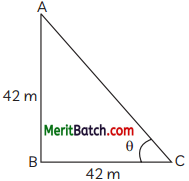Then, in ∆ABC,
tan θ = $$\frac{AB}{BC}$$ = $$\frac{42}{42}$$ = 1
tan θ = tan 45°
⇒ θ = 45°

OR
Let C be the point of observation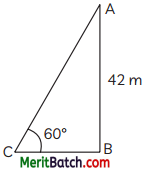tan 60° = $$\frac{AB}{BC}$$
$$\sqrt{3}$$ = $$\frac{42}{BC}$$
BC = $$\frac{42}{\sqrt{3}}$$ × $$\frac{\sqrt{3}}{\sqrt{3}}$$ = 14$$\sqrt{3}$$ m

(B) Let PQ be a tower and RQ be its shadow of length 20 m.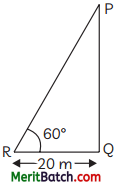In ∆PQR,
tan 60° = $$\frac{PQ}{QR}$$
$$\sqrt{3}$$ = $$\frac{PQ}{20}$$
PQ = 20$$\sqrt{3}$$ m

(C) If ratio of height of pole and length of shadow is 1 : 1, then they are equal in length.
tan θ = $$\frac{1}{1}$$ = 1
θ = 45°

Question 37.
Ashok is trying to find the height of a tower near his house. He is using the properties of similar triangles. The height of Ashok’s house is 20 m and casts a shadow of length 10 m at the ground. At the same time, the tower casts 50 m long shadow on the ground and the house of Prem casts 20 m shadow on the ground.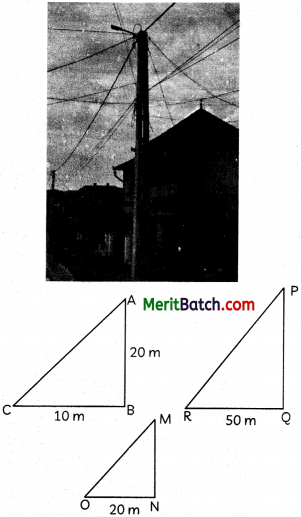Based on the above information, answer the following questions:
(A) What is the height of the tower? And find, the height of Prem’s house.
OR
When the tower casts a shadow of 40 m, same time what will be the length of the shadow of Prem’s house?
(B) What will be the length of the shadow of the tower when Ashok’s house casts a shadow 12 m long?
(C) When the tower casts a shadow of 40 m, same time what will be the length of the shadow of Ashok’s house?
(A) Since, the sun’s elevation is same
∴ ∆ABC ~ ∆PQR
as ∠ABC = ∠PQR = 90°
and ∠ACB = ∠PRQ
$$\frac{AB}{PQ}$$ = $$\frac{BC}{RQ}$$
(Corresponding sides of similar As)
∴ $$\frac{20}{PQ}$$ = $$\frac{10}{50}$$
∴ PQ =100 m
∆ABC ~ ∆MNO
∴ $$\frac{AB}{MN}$$ = $$\frac{BC}{ON}$$
∴ $$\frac{20}{MN}$$ = $$\frac{10}{20}$$
MN = 40
OR
Height of tower = 100 m
Shadow of tower = 40 m
Height of Prem’s house = 40 m
Shadow of Prem’s house = x
By property of similar triangle
∴ $$\frac{100}{40}$$ = $$\frac{40}{x}$$
x = $$\frac{1600}{100}$$ = 16 m

(B) If the Length of shadow of Ashok’s house is 12 m, then by property of similar triangles
$$\frac{20}{12}$$ = $$\frac{100}{x}$$
where x is the the length of the shadow of the tower
x = 60 m

(C) (d) 8 m
Explanation: If tower is making a shadow of 40 m, and its height is 100 m (as calculated)
Height of Ashok’s house is: 20 m
∴ Let, length of its shadow be y.
∴ By property of similar triangles
$$\frac{100}{40}$$ = $$\frac{20}{y}$$
y = 8 mQuestion 38.
An agency has decided to install customized playground equipments at various colony parks. For that they decided to study the age – group of children playing in a park of the particular colony. The classification of children according to their ages, playing in the park is shown in the following table: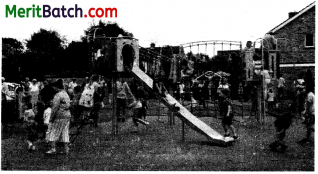Age group of children (in years) Number of children 6-8 43 8-10 58 10-12 70 12-14 42 14-16 27

Based on the above information, answer the following questions:
(A) Which age – group has the maximum number of children?
(B) What is the lower limit of the modal class?
(C) What is the modal age (in years) of children?
OR
If the mean of numbers 2, a, 6 and 7 is 15 and the mean of numbers 6, 18, 1, a, b, is 20, then find the value of b.
(A) There are 70 children in age group 10-12.
(B) As modal class is 10-12.
(C) Here, Modal class 10-12
l = 10, f0 = 58, f1 = 70, f2 = 42, h = 2
∴ Mode = $$l+\left(\frac{f_1-f_0}{2 f_1-f_0-f_2}\right) \times h$$
= $$10+\left(\frac{70-58}{140-58-42}\right) \times 2$$
= 10 + $$\frac{24}{40}$$
= 10 + 0.6
= 10.6

OR

Given: Mean of 2, a, 6 and 7 is 15.
∴ $$\frac{2+a+6+7}{4}$$ = 15
15 + a = 60
a = 45
Also, mean of 6, 18, 1, a and b is 20.
$$\frac{6+18+1+a+b}{5}$$ = 20
25 + a + b = 100
25 + 45 + b = 100
b = 100 – 70
b = 30
Hence, the value of b is 30.